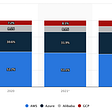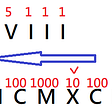# [LeetCode]#371. Sum of Two Integers

`Input: a = 1, b = 2Output: 3`
`Input: a = -2, b = 3Output: 1`
`class Solution:    def getSum(self, a, b):mask = 0xffffffff        sum = (a ^ b) & mask        carry = a & b        while carry!=0:            a = sum            b = (carry << 1) & mask            sum = (a ^ b) & mask            carry = a & b        if sum & 0x80000000:            sum -= 0x100000000        return sum`

## More from Fatboy Slim

Interesting in any computer science.

Love podcasts or audiobooks? Learn on the go with our new app.

## Building a hash cracking tool using Python## The Pythonic Way## Time Complexities By Insertion Sort , Merge Sort And Quick Sorts## Why are most companies using cloud computing? ☁️## Deep dive into AWS for developers | Part2## Non-Blocking Java — Beyond the Hype## Fatboy Slim

Interesting in any computer science.

## 13. Roman to Integer## Two Sum | Interview Question## [LeetCode]#2119. A Number After a Double Reversal